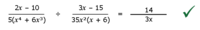# Dividing Fractions

#### Algebraneophyte

##### New memberHi Guys.

I got this one correct, but unsure on my full working. This is as follows:

When dividing fractions we cancel down parallel and then switch one and multiply.

2(x-5) / (5(x^4 + 6x^3) ÷ 3(x-5) / 35(x^3+6x^2)

(x^4+6x^3) ÷ (x^3+6x^2) *does this equal x+x or x?* *(I think it is one factor of x we take out , but if you could explain why precisely that would be great)*

35/5 = 7/1

So we have: (2/x) ÷ (3/7) = (2/x) X (7/3) = 14/3x

#### ISTER_REG

##### New member
$$\displaystyle \frac{2x-10}{5(x^4+6x^3)} \div \frac{3x-15}{35x^2(x+6)}$$

$$\displaystyle \frac{2(x-5)}{5(x^4+6x^3)} \cdot \frac{35x^2(x+6)}{3(x-5)}$$

$$\displaystyle \frac{70}{15}\frac{x^2(x+6)}{x^2(x^2+6x)}$$

$$\displaystyle \frac{14}{3} \frac{(x+6)}{x(x+6)}$$

#### Harry_the_cat

##### Elite Member
View attachment 26936

Hi Guys.

I got this one correct, but unsure on my full working. This is as follows:

When dividing fractions we cancel down parallel and then switch one and multiply.

2(x-5) / (5(x^4 + 6x^3) ÷ 3(x-5) / 35(x^3+6x^2)

(x^4+6x^3) ÷ (x^3+6x^2) *does this equal x+x or x?* *(I think it is one factor of x we take out , but if you could explain why precisely that would be great)*

35/5 = 7/1

So we have: (2/x) ÷ (3/7) = (2/x) X (7/3) = 14/3x
You mention (x^4+6x^3) ÷ (x^3+6x^2). In this problem, it is the other way around, but to answer your question...
You must always cancel FACTORS.
To get x+x, I think you are attempting to cancel the x^3 into the x^4 and the 6x^2 into the 6x^3. You can't do that because the x^3 and the 6x^2 are NOT factors. (Factors are expressions which are multiplied together.)
To be able to cancel factors, you first need to factorise the top and bottom.
This becomes x^3(x+6) ÷ x^2(x+6). The (x+6) can be cancelled because they are factors. This leaves x^3 ÷ x^2 which is x (after cancelling the factor x^2).

#### Algebraneophyte

##### New member
Thank you very much guys. I will practice more questions before moving to Algebra with graphing.

•Harry_the_cat